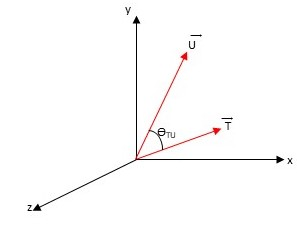# Problem: The figure shows two vectors T_vec and U_vec separated by an angle theta_TU. (Figure 1) You are given that vec{T} = (3,1,0), vec{U} = (2,4,0), and vec{T} × vec{U} = vec{V}. Find the sine of the angle between T_vec and U_vec.

###### FREE Expert Solution

Vector Magnitude:

$\overline{)|\stackrel{⇀}{\mathbit{A}}|{=}\sqrt{{{\mathbit{A}}_{\mathbit{x}}}^{2}+{{\mathbit{A}}_{\mathbit{y}}}^{2}+{{\mathbit{A}}_{\mathbit{z}}}^{2}}}$

$\overline{){\mathbf{|}}{\mathbf{V}}{\mathbf{|}}{\mathbf{=}}{\mathbf{|}}{\mathbf{T}}{\mathbf{|}}{\mathbf{|}}{\mathbf{U}}{\mathbf{|}}{\mathbf{s}}{\mathbf{i}}{\mathbf{n}}{\mathbf{\theta }}}$

|T| = (Tx2 + Ty2 + Tz2) = (32 + 12 + 02)(1/2) = 10(1/2)

93% (413 ratings)###### Problem Details

The figure shows two vectors T_vec and U_vec separated by an angle theta_TU. (Figure 1) You are given that vec{T} = (3,1,0), vec{U} = (2,4,0), and vec{T} × vec{U} = vec{V}.

Find the sine of the angle between T_vec and U_vec.